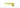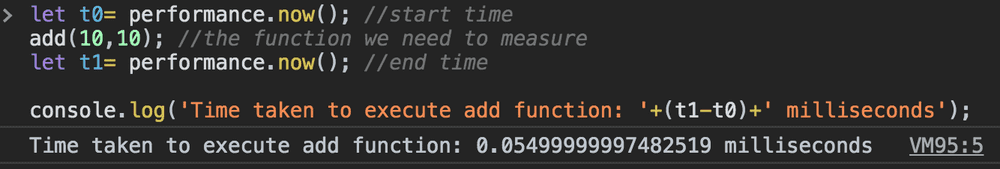# How to Measure the Function Execution time in JavaScriptIn this tutorial, we are going to learn about measuring the function execution time in JavaScript.

Consider we have an `add()` function which is used to add the two numbers and returns their sum.

``````function add(a,b){
return a+b;
}

Now we need to measure, how much time it takes to execute this `add()` function by using `performance.now()` method.

## Measuring function execution time

The `performance.now()` method returns a high-resolution timestamp in milliseconds.

Example:

``````let t0= performance.now(); //start time
add(10,10); //the function we need to measure
let t1= performance.now(); //end time

console.log('Time taken to execute add function:'+ (t1-t0) +' milliseconds');``````

In the above code, we have invoked `add()` function in between `t0`(start time) and `t1`(end time) ,inside `console.log()` we subtracted `t1-t0` so that we can get the `add()` function execution time in milliseconds.If you want to measure the time in seconds instead of milliseconds we can do that by dividing milliseconds with `1000`.

``console.log('Time taken to execute add function:'+ (t1-t0)/1000 +' seconds');``

## Top Udemy Courses##### JavaScript - The Complete Guide 2020 (Beginner + Advanced)
45,614 students enrolled
52 hours of video content
View Course##### React - The Complete Guide (incl Hooks, React Router, Redux)
284,472 students enrolled
40 hours of video content
View Course##### Vue JS 2 - The Complete Guide (incl. Vue Router & Vuex)
130,921 students enrolled
21 hours of video content
View Course